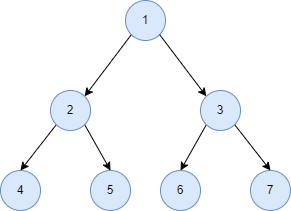# Inorder Traversal of a Tree in Python

Fariba Laiq Jul 01, 2022

A Tree is a hierarchical data structure that consists of nodes connected by edges. Traversing a tree means visiting every node of the tree exactly once.

We traverse the tree for different purposes like displaying the nodes, finding the largest and smallest node, searching, sorting, etc. In this article, we will learn and implement the `inorder` traversal of a tree in Python.

## Inorder Traversal of a Tree

`Inorder` traversal is a kind of depth-first traversal. Suppose we have the following tree.If we apply `inorder` traversal, we will follow the steps below for each node.

1. First, we should visit all the nodes of the left subtree.
2. Then, we visit the parent node.
3. Then, we visit all the nodes in the right subtree.

We will get the nodes in the order `4, 2, 5, 1, 6, 3, 7`.

## Inorder Tree Traversal Implementation in Python

There are two ways to implement the `inorder` traversal in Python. The recursive and the iterative approach.

### Recursive Approach

The recursive approach is easy to implement and understand. In the following code, we have created a class `Node` as a data structure to store the tree.

Each node consists of a value, its left and right child. The `inorder` traversal will recursively work for the left and right subtrees.

For every node, the `inorder` traversal will be performed by visiting its left node, the parent, and the right node.

Example Code:

``````class Node:
def __init__(self, value):
self.left = None
self.right = None
self.val = value
def inorder(root):
if root:
inorder(root.left)
print(str(root.val))
inorder(root.right)
root = Node(1)
root.left = Node(2)
root.right = Node(3)
root.left.left = Node(4)
root.left.right = Node(5)
root.right.left = Node(6)
root.right.right = Node(7)
print("Inorder traversal of the Tree")
inorder(root)
``````

Output:

``````Inorder traversal of the Tree
4
2
5
1
6
3
7
``````

### Iterative Approach

In an iterative approach, we must maintain a `stack` to store the nodes we will visit later. We created the class `Node` in the following code, just like before.

We have created an empty stack and started from the root node by making it the current node. If the current node exists, we will push it to the stack and go to its left node.

Else, if the node does not exist, we will pop an element from the stack and print it. When no left node exists, we will go to the right node by making it the current node.

We will repeat the same procedure iteratively until both the stack and the current element is empty.

Example Code:

``````from collections import deque
class Node:
def __init__(self, value):
self.left = None
self.right = None
self.val = value
def inorder(root):
stack = deque()
curr = root
while stack or curr:
if curr:
stack.append(curr)
curr = curr.left
else:
curr = stack.pop()
print(curr.val)
curr = curr.right
root = Node(1)
root.left = Node(2)
root.right = Node(3)
root.left.left = Node(4)
root.left.right = Node(5)
root.right.left = Node(6)
root.right.right = Node(7)
print("Inorder traversal of the Tree")
inorder(root)
``````

Output:

``````Inorder traversal of the Tree
4
2
5
1
6
3
7
``````
Author: Fariba Laiq

I am Fariba Laiq from Pakistan. An android app developer, technical content writer, and coding instructor. Writing has always been one of my passions. I love to learn, implement and convey my knowledge to others.